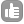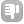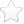0how T1 and T2 relaxation time depend on nmr signal? asked Oct 21 '13 at 00:591Well, if nobody else answers... T2 is related to NMR spectrum width in solids and viscous liquids, spectrum half-width is approx. equal to 2/T2. I believe T1 is unrelated to the spectral shape. But the spectra one gets are depending on T1 and T2 severily. If relaxation delay in experiment is short (usually comparing to 5*T1), the signal will be weak. If one shortens T2 (say, dissolving a paramagnetic component in a liquid sample), the lines will be broaden. answered Oct 23 '13 at 02:03asbest 110The question sounds backwards. Take a look at the classic "Pulse and Fourier Transform NMR" by Ferrer and Becker T1 and T2 are time constants that come from the phenomenological bloch equations. T1 determines how much signal we detect. Waiting 5*T1 allows the spins to return to thermal equilibrium before the next perturbation. T2 is the time constant for decay in the transverse plane. In practice, T1 effects the signal more than T2. T2 can be used to find M(t=0) if you want to quantify signal amplitude. Relaxation enhancement agents such as paramagnetic species can effect T1 and T2 differently and are more commonly used to decrease T1 so we do not have to wait. e.g., H2O has T1=T2=2.5s on my instrument (100MHz). When I dope with 1ppm CuSO4 * 5H2O I measure T1=0.28 T2=0.25 now 5*T1 is a reasonable 1s wait time :) answered Oct 29 '13 at 07:39